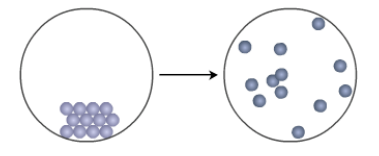# Problem: The diagram below depicts the spontaneous sublimation of I2 (s) at 25°C and 1 atm: Accordingly, ΔSsys is ______ , ΔSsurr is _______, and ΔStotal is _______ for this transformation. (a) positive, positive, positive (b) positive, negative, positive (c) negative, positive, positive (d) positive, positive, negative (e) negative, positive, negative

###### FREE Expert Solution
88% (394 ratings)
###### Problem Details

The diagram below depicts the spontaneous sublimation of I(s) at 25°C and 1 atm:

Accordingly, ΔSsys is ______ , ΔSsurr is _______, and ΔStotal is _______ for this transformation.

(a) positive, positive, positive

(b) positive, negative, positive

(c) negative, positive, positive

(d) positive, positive, negative

(e) negative, positive, negativeWhat scientific concept do you need to know in order to solve this problem?

Our tutors have indicated that to solve this problem you will need to apply the Second and Third Laws of Thermodynamics concept. If you need more Second and Third Laws of Thermodynamics practice, you can also practice Second and Third Laws of Thermodynamics practice problems.

What is the difficulty of this problem?

Our tutors rated the difficulty ofThe diagram below depicts the spontaneous sublimation of I2 ...as medium difficulty.

How long does this problem take to solve?

Our expert Chemistry tutor, Dasha took 2 minutes and 4 seconds to solve this problem. You can follow their steps in the video explanation above.

What professor is this problem relevant for?

Based on our data, we think this problem is relevant for Professor Rowland's class at OLE MISS.